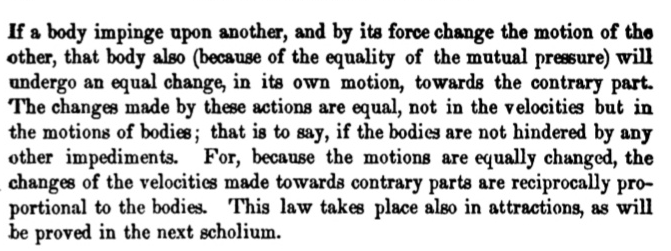Newton's Laws of Dynamics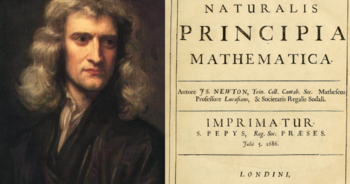Ancient Philosophers were quite confused about the motion of objects. What's the cause of motion? What is the nature of the cause? This confusion about objects persisted until  17th century when Galileo Galilei and Isaac Newton developed a approach to understanding those motions which we call 'Classical Mechanics'.  Newton presented his three laws of motion in 1687 AD in his book, 'Philosophiae Naturalis Principia Mathematica', usually called the Principia. His work is considered as one of the most revolutionizing and significant contributions in  Physics. This laws comes under a branch of Physics called 'Dynamics' which studies cause of motion.

Newton First Law of Motion﻿

Before Newton, Galileo discovered the principle of inertia which  states that all external impediments removed, a heavy body on a spherical surface concentric with the earth will maintain itself in that state in which it has been; if placed in movement towards the west, it will maintain itself in that movement. He discovered in the form of circular inertia rather rectilinear inertia which was done by Newton. So, Newton's First law is mere restatement of Galilean principle of inertia. That's why the Newton's first law is also considered as Law of Inertia.

Newton's First Law of Motion is stated as," A body will continue to be at the original state of motion which is rest or constant velocity unless it is acted by external force that  will change its original state."

Since, the resultant force on the body should be  zero,we can also say that to be in rest or constant velocity, the body which is considered as a point particle, should be in the state of equilibrium whether that is static or dynamic equilibrium.

Below is the original interpretation given by Newton in his book principia,Beside this the Newton's first law of motion is also the assertion that the inertial system exist. Each observer observing the objects moving defines a reference frame which is relative to the observer. A reference frame requires a coordinate system and a set of clocks, which enable an observer to measure positions, velocities, and accelerations in his or her particular reference frame. Observers in different reference frames may measure different velocities or accelerations. Suppose you are a passenger held by a seat belt in a bus travelling at certain velocity and a box, ahead of you is at rest on the floor. When the brakes are applied, the box will be pushed forward, but there wasn't any apparent force acting on the box which of course violates the Newton's first law of motion. But if a girl from footpath is somehow watching the box, the box will move at the initial velocity of bus in the absence of friction and she detects no violation of Newton's first law. So, there is a special frame of reference in which the Newton's laws holds, which is called Inertial Frame of Reference. This frame contains the body which is at rest or constant velocity.

A wonderful Insight of  Frame of Reference is given by this video:

Newton's Second Law of Motion

This law is the most fundamental law of nature that gives us insight about how the motion changes when force is applied to body. Newton's Second Law of Motion is stated as 'If a net external force acts on a body, the body accelerates. The mass of the body times the acceleration vector of the body equals the net force vector. The direction of acceleration is the same as the direction of the net force.'This law is normally stated as the rate of change of momentum is force which is the  original statement of Newton in his book Principia. Momentum quantifies the motion of  a body and is the product of mass of the body  and its velocity.Then,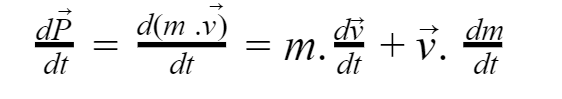We take Newtonian approximation that the mass of the body is constant but in the case of rocket, the mass is also changing due to which  we have to know how mass changes with velocity. Then our equation becomes,So, Newton's Second law can also be stated as, 'The resultant force acting on a particle equals the time rate of change of momentum of the particle.'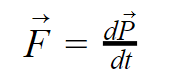Note the above equation is vector equation, its cartesian coordinates is written as,Fig: change in velocity in all directions.

Newton in his Principia interpreted his second law as follows:This law also directly gives us a pseudo work equation for complex systems that can be found here.

Newton's Third Law of Motion

We normally state this law as, 'To every action force, there is always an equal and opposite reaction force.' It doesn't interpret the alignment of the forces with along the line joining two bodies.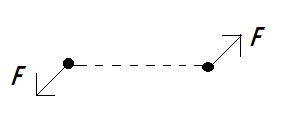Fig: Forces which are not along the line of two bodies.

These forces are not considered as pair forces as they are not along the line joining two bodies. That's why it is considered as weak form of Newton's third law.

A strong form of Newton's third law is stated as, 'Force by particle A on another particle B and force by particle B on particle A which are along the line joining the particles at any instant are equal in magnitude and opposite in direction.' Newton may or may not actually have said this, but he tacitly assumed it.Fig: Forces which are along the line joining the bodies.

We also refer to these force as action-reaction pair. This doesn't imply any cause and effect relationship between them but simply implies that they are equal and opposite.

It is very helpful to remember that any two forces acting on same object cannot be a action-reaction pair because third law pairs are always of the form of a force from A to B and another force from B to A. For example, when you stand stationary on the ground, there is a gravitational force downward on you and a normal force upward from the ground. These forces are equal in magnitude and opposite in direction. Still, they are not a action-reaction pair because these forces are in fact not always equal in magnitude. For example, if you jump, then while you are accelerating upward, the normal force on you from the ground is greater than the gravitational force.

The third law pair for the gravitational force on you is the gravitational force that you exert on the Earth and for the normal force that the ground exerts on you is the normal force that you exert on the ground. The Centrifugal and Coriolis forces do not have third law pairs as they only appear in accelerating frames, where Newton's laws require modification.

This law also essentially postulate that momentum is conserved.

Let, the bodies have mass m1 and m2 . Similarly, forces exerted by each other are F1,F2 and their velocities v1 and v2 .Then, total momentum in the system is,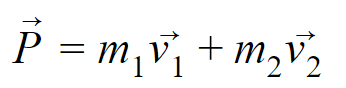Now, calculating rate of change of momentum,Since, F1 = -F2 we get,Therefore, momentum conservation is a consequence of Newton's third law, but angular momentum is also conserved. For forces to conserve angular momentum, they need to obey another condition on top of Newton's third law, which is that both forces in any third-law pair have the same moment arm about any axis.

Newton's original interpretation is given below,